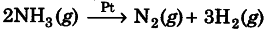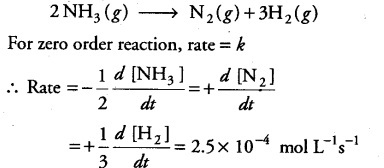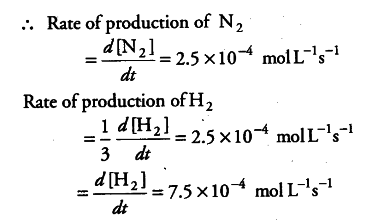# What are the rates of production of \${ N }_{ 2 }\$ and \${ H }_{ 2 }\$?

The decomposition of N{ H }_{ 3 } on platinum surface,is a zero order with k = 2.5x 10-4 mol L-1s-1
What are the rates of production of { N }_{ 2 } and { H }_{ 2 }?